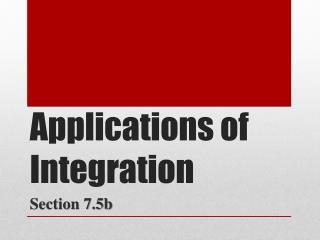Download PresentationApplications of Integration

Loading in 2 Seconds...

# Applications of Integration - PowerPoint PPT Presentation

Applications of Integration. Section 7.5b. Fluid Force and Fluid Pressure. We build dams wider at the bottom than at the top. Why?. What does the greater pressure at the bottom of the d am depend upon?. Only on the type of liquid and how far below theI am the owner, or an agent authorized to act on behalf of the owner, of the copyrighted work described.
Download Presentation## Applications of Integration

An Image/Link below is provided (as is) to download presentation

Download Policy: Content on the Website is provided to you AS IS for your information and personal use and may not be sold / licensed / shared on other websites without getting consent from its author.While downloading, if for some reason you are not able to download a presentation, the publisher may have deleted the file from their server.

- - - - - - - - - - - - - - - - - - - - - - - - - - E N D - - - - - - - - - - - - - - - - - - - - - - - - - -
Presentation Transcript
1. Applications of Integration Section 7.5b

2. Fluid Force and Fluid Pressure We build dams wider at the bottom than at the top. Why? What does the greater pressure at the bottom of the dam depend upon? • Only on the type of liquid and how far below the • surface the point lies (not on how much liquid the • dam is holding back) Read about “The Great Molasses Flood of 1919” at the top of p.404…

3. Fluid Force and Fluid Pressure Given that the tank was full of molasses weighing , what was the total force exerted by the molasses on the bottom of the tank at the time it ruptured? In any liquid, the fluid pressurep (force per unit area) at depth h is where w is the weight-density (weight per unit volume of the given liquid)

4. Fluid Force and Fluid Pressure Given that the tank was full of molasses weighing , what was the total force exerted by the molasses on the bottom of the tank at the time it ruptured? At the bottom of this tank, the pressure from the molasses: The area of the base of the tank: Total force on the base:

5. Fluid Force and Fluid Pressure (b) What was the total force against the bottom foot-wide band of the tank wall? Partition the band into narrower bands of width , depth Pressure at this depth: Force against each narrow band = (pressure)(area):

6. Fluid Force and Fluid Pressure (b) What was the total force against the bottom foot-wide band of the tank wall? Force against each narrow band = (pressure)(area): Summing these forces for the entire partition:

7. Fluid Force and Fluid Pressure A rectangular milk carton measures 3.75in by 3.75in at the base and is 7.75in tall. Find the force of the milk (weighing ) on one side when the carton is full. Force equals pressure applied over an area:

8. Normal Probabilities In mathematics, probabilities are represented as areas, which is where integrals come into play… Definition: Probability Density Function (pdf) A probability density function is a function with domain all reals such that for all x and Then the probability associated with an interval is

9. Normal Probabilities By far the most useful pdf is the normal kind… Definition: Normal Probability Density Function (pdf) A normal probability density function (Gaussian curve) for a population with mean and standard deviation is

10. Normal Probabilities And an extremely valuable rule… The 68-95-99.7 Rule for Normal Distributions Given a normal curve, • 68% of the area will lie within of the mean • 95% of the area will lie within of the mean • 99.7% of the area will lie within of the mean

11. Normal Probabilities Suppose that frozen spinach boxes marked as “10 ounces”of spinach have a mean weight of 10.3 ounces and a standard deviation of 0.2 ounce. The pdf: Graph this function in [9, 11.5] by [-1, 2.5] Does the function have the expected shape?

12. Normal Probabilities Suppose that frozen spinach boxes marked as “10 ounces”of spinach have a mean weight of 10.3 ounces and a standard deviation of 0.2 ounce. What percentage of all such spinach boxes can be expected to weigh between 10 and 11 ounces? The probability that a randomly chosen spinach box weighs between 10 and 11 ounces is the area under the curve from 10 to 11. Find numerically: There is approximately a 93.296% chance that a box will weigh between 10 and 11 ounces.

13. Normal Probabilities Suppose that frozen spinach boxes marked as “10 ounces”of spinach have a mean weight of 10.3 ounces and a standard deviation of 0.2 ounce. (b) What percentage would we expect to weigh less than 10 ounces? We need the area under the curve to the left of x = 10… Because of the asymptote, we use x = 9 as an approximation for the lower bound: There is approximately a 6.681% chance that a box will weigh less than 10 ounces.

14. Normal Probabilities Suppose that frozen spinach boxes marked as “10 ounces”of spinach have a mean weight of 10.3 ounces and a standard deviation of 0.2 ounce. (c) What is the probability that a box weight exactly 10 ounces? This would be the integral from 10 to 10, which is zero. Huh??? Remember that we are assuming a continuous, unbroken interval of possible weights, and 10 is but one of an infinite number of them…